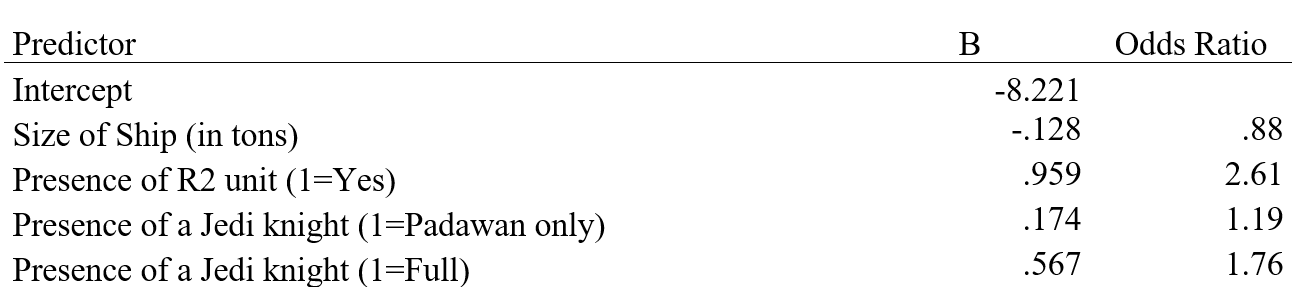# Guidelines for writing up three types of odds ratios

Odds ratios have a unique part to play in describing the effects of logistic regression models. But that doesn’t mean they’re easy to communicate to an audience who is likely to misinterpret them. So writing up your odds ratios has to be done with care.

Precise word choice is vital. So I give you the precise language I use when writing up the results of a logistic regression, as well as an explanation.

### The logistic regression example

I’ve borrowed an example from my free Craft of Statistical analysis webinar: Understanding Probability, Odds, and Odds Ratios. If you need a refresher on what any of these terms means, I’d suggest watching it. Imagine you’ve just run a logistic regression and get the following results:In this example, we’re exploring the effect of a few predictors on the odds of successfully navigating an asteroid field. Although the odds may, in general, be small, we’d like to see which variables affect those odds.

We’ve entered three predictors:

1. “Size of Ship” is numerical.
2. “Presence of an R2 Unit”* is binary. It is dummy coded so that Yes=1 and No = 0.
3. “Presence of a Jedi” has three categories: None; Yes, Padawan only; and Yes, Jedi Knight with or without a Padawan. We need two dummy variables to measure the effect of the three categories.

### Guidelines of the write-up

I’ll give you examples of how you’d write each one, but first I want to point out a few guidelines I suggest following in order to be both accurate and clear.

1. Always use the word “odds,” not “likely.” (And never probability when you mean odds. They’re not the same measure). While in everyday English, it makes sense to substitute likely for odds, most readers will interpret likely as a probability, not an odds. While both probability and odds measure how likely the outcome is, this is a situation where lack of precision in your language will lead to misinterpretation.

It’s like talking about the temperature in degrees in the US, without specifying that you mean degrees Celsius. It’s not wrong to say degrees, it’s just imprecise and therefore likely to be misinterpreted.

2. Always make it crystal clear which outcome category you’re predicting the odds of.

3. If your data are observational, not experimental, your predictors will not be independent. So you’ll have to always include the caveat that each odds ratio is the effect of that predictor, after adjusting for the effects of the other predictors.

Since it would unethical to randomly assign Jedi to ships unlikely to make it through an asteroid field, we’ll assume these results came from observational data.

4. There are two ways to describe an odds ratio. One is simply “OR times the odds.” The other is as a (OR-1)*100% increase.

The former is easier to understand if the Odds Ratio is 2 or more. I use this for the R2 unit odds ratio.

The latter is easier to understand if the odds ratio is between 1 and 2. I use this for the Jedi odds ratios. The latter is also easier to understand if the odds ratio is below 1, but the negative percentage can be described as a decrease. I use this for the Size of the Ship odds ratio.

### Examples of writing up each odds ratio

#### Binary predictor, odds ratio > 2:

After adjusting for size of ship and presence of a Jedi (Padawan or Knight), ships with an R2 unit have odds of successfully navigating an asteroid field that are 2.61 times those of ships without an R2 unit.

#### Numerical predictor, odds ratio < 1:

After adjusting for the presence of an R2 unit and a Jedi (Padawan or Knight), larger ships show a decreased odds of successfully navigating an asteroid field. For each additional ton of the size of the shop, the odds of success decrease by 12%.

#### Multicategory predictor, odds between 1 and 2:

After adjusting for size of ship and presence of an R2 unit, having a Jedi aboard is associated with increased odds of successfully navigating an asteroid field, though the effect appears larger for a Jedi Knight than it is for a Jedi Padawan. Compared to ships with no Jedi at all, having only a Padawan aboard is associated with odds that are 19% higher and having a Knight aboard is associated with odds that are 76% higher.

*Yes, this is one of those effects that is so obvious it may seem silly to test. We all know who is really flying the ship.Understanding Probability, Odds, and Odds Ratios in Logistic Regression
Despite the way the terms are used in common English, odds and probability are not interchangeable. Join us to see how they differ, what each one means, and how to tame that tricky beast: Odds Ratios.